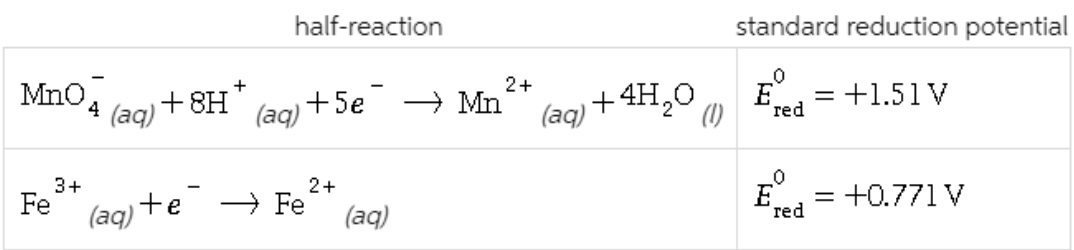# Problem: A chemist designs a galvanic cell that uses these two half-reactions:Write a balanced equation for the half-reaction that happens at the cathode. Write a balanced equation for the half-reaction that happens at the anode.Write a balanced equation for the overall reaction that powers the cell. Be sure the reaction is spontaneous as written.Do you have enough information to calculate the cell voltage under standard conditions?If you said it was possible to calculate the cell voltage, do so and enter your answer here. Round your answer to 2 significant digits.

###### FREE Expert Solution

Based on the half-reactions shown, a chemist designs a galvanic cell. We are asked to write the balanced equations for the half-reactions at the cathode, anode, and overall reaction. And additionally, to calculate the cell voltage if possible.

Assuming the reaction is under acidic conditions, we will follow the following steps to balance the reactions:

Step 1: Separate the whole reaction into two half-reactions

Step 2: Balance the non-hydrogen and non-oxygen elements first

Step 3: Balance oxygen by adding H2O to the side that needs oxygen (1 O: 1 H2O)

Step 4: Balance hydrogen by adding H+ to the side that needs hydrogen (1 H: 1 H+)

88% (208 ratings)###### Problem Details

A chemist designs a galvanic cell that uses these two half-reactions:Write a balanced equation for the half-reaction that happens at the cathode.

Write a balanced equation for the half-reaction that happens at the anode.

Write a balanced equation for the overall reaction that powers the cell. Be sure the reaction is spontaneous as written.

Do you have enough information to calculate the cell voltage under standard conditions?

If you said it was possible to calculate the cell voltage, do so and enter your answer here. Round your answer to 2 significant digits.

Frequently Asked Questions

What scientific concept do you need to know in order to solve this problem?

Our tutors have indicated that to solve this problem you will need to apply the Cell Potential concept. If you need more Cell Potential practice, you can also practice Cell Potential practice problems.

What professor is this problem relevant for?

Based on our data, we think this problem is relevant for Professor Metlitsky's class at BROOKLYN CUNY.